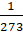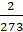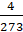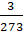Daily Practice Problems
Class 11 Chemistry
States of MatterQuestion1.

Derive numerical values of R in L atm K-1 mol-1 , J K-1 mol-1 , calorie K-1 mol-1.

Question2.

A gas occupies 20 litre under a pressure of 1 atmosphere. What will be its volume if the pressure is increased to 2 atmosphere? (Temperature is constant)

Question3.

A gas occupies 600 ml at 27 °C and 1 atm. What will be the volume at 127 °C if the pressure is kept constant?

Question4.

Gas Cylinder containing cooking gas can withstand a pressure of 14.9 atm. The pressure gauge of the cylinder indicates 12 atm at 27 °C. Due to a sudden fire in the building, the temperature starts rising. At what temperature will the cylinder explode?

Question5.

A gas having volume 1000 ml at -73 °C and 2 atm is heated to 123 °C and the pressure is decreased to 0.5 atm. What will be final volume?

Question6.

3.7 g of a gas at 25 °C occupies the same volume as 0.184 g of H2 at 17 °C and at the same pressure. Find the molar mass of gas?

Question7.

What is the pressure of HCl gas at -40 °C if its density is 8.0 kg m-3 ? (R = 8.314 J K-1 mol-1)

Question8.

A certain quantity of a gas occupies 100 mL when collected over water at 15°C and 750 mm pressure. It occupies 91.9 mL in dry state at NTP. What will be the aqueous vapour pressure at 15 °C?

Question9.

A balloon of diameter 20 m weighs 100 kg. Calculate its payload if it is filled with He at 1.0 atm and 27 °C. Density of air is 1.2 kg m-3. (R = 0.082 dm3  atm K-1 mol-1)

Question10.

Coefficient of volume expansion of a gas is:

1)2)3)4)Question11.

A 10 L flask contains 0.2 mole of methane, 0.3 mole of hydrogen and 0.4 mole of nitrogen at 25°C. What is the partial pressure of each component and what is the pressure inside the flask?

Question12.

What is Viscosity coefficient? Give its SI unit.

Question13.

The van der Waals' constant' a' for the gases O2, N2, NH3 and CH4 are 1.36, 1.39, 4.17 and 2.253 respectively, the gas which can be most easily liquefied is:

1) O2

2) N2

3) NH3

4) CH4

Question14.

Why Surface tension decreases as temperature increases?

Question15.

What is Boyle point?

Question16.

Give the postulates of Kinetic theory of gases in brief.

Question17.

Give the physical properties of gases.

Question18.

Which elements exists as gases under normal conditions?

Question19.

Why viscosity of liquids decreases as temperature increases?

Question20.

State Dalton’s Law of Partial Pressures.

***********